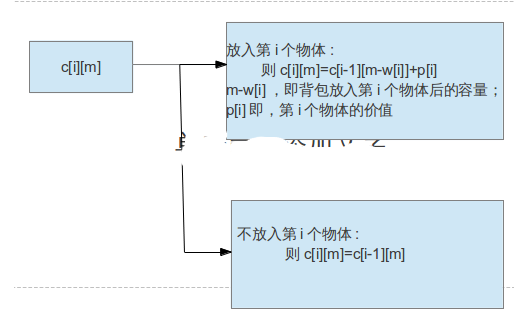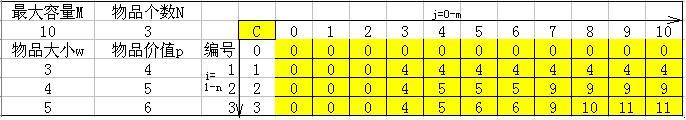从01背包问题理解动态规划---初体验 转

r
ranjiewen

2019独角兽企业重金招聘Python工程师标准>>>01背包问题具体例子：假设现有容量10kg的背包，另外有3个物品，分别为a1，a2，a3。物品a1重量为3kg，价值为4；物品a2重量为4kg，价值为5；物品a3重量为5kg，价值为6。将哪些物品放入背包可使得背包中的总价值最大？

这个问题有两种解法，动态规划和贪婪算法。本文仅涉及动态规划。

先不套用动态规划的具体定义，试着想，碰见这种题目，怎么解决?

首先想到的，一般是穷举法，一个一个地试，对于数目小的例子适用，如果容量增大，物品增多，这种方法就无用武之地了。

其次，可以先把价值最大的物体放入，这已经是贪婪算法的雏形了。如果不添加某些特定条件，结果未必可行。

最后，就是动态规划的思路了。先将原始问题一般化，欲求背包能够获得的总价值，即欲求前i个物体放入容量为m（kg）背包的最大价值c[i][m]——使用一个数组来存储最大价值，当m取10，i取3时，即原始问题了。而前i个物体放入容量为m（kg）的背包，又可以转化成前(i-1)个物体放入背包的问题。下面使用数学表达式描述它们两者之间的具体关系。

表达式中各个符号的具体含义。

w[i] :  第i个物体的重量；

p[i] : 第i个物体的价值；

c[i][m] ： 前i个物体放入容量为m的背包的最大价值；

c[i-1][m] ： 前i-1个物体放入容量为m的背包的最大价值；

c[i-1][m-w[i]] ： 前i-1个物体放入容量为m-w[i]的背包的最大价值；

由此可得：

c[i][m]=max{c[i-1][m-w[i]]+pi , c[i-1][m]}（下图将给出更具体的解释）思路厘清后，开始编程序，C语言代码如下所示。

``````#include <stdio.h>
int c={0};

void knap(int m,int n){

int i,j,w,p;
for(i=1;i<n+1;i++)
scanf("%d,%d",&w[i],&p[i]);
for(j=0;j<m+1;j++)
for(i=0;i<n+1;i++)
{
if(j<w[i])
{
c[i][j]=c[i-1][j];
continue;
}else if(c[i-1][j-w[i]]+p[i]>c[i-1][j])
c[i][j]=c[i-1][j-w[i]]+p[i];
else
c[i][j]=c[i-1][j];
}

}

int main(){
int m,n;int i,j;
printf("input the max capacity and the number of the goods:\n");
scanf("%d,%d",&m,&n);
printf("Input each one(weight and value):\n");
knap(m,n);
printf("\n");
for(i=0;i<=n;i++)
for(j=0;j<=m;j++)
{
printf("%4d",c[i][j]);
if(m==j) printf("\n");
}
}``````
``代码中，红色字体部分是自己写的，其余的参照了这篇博客http://blog.sina.com.cn/s/blog_6dcd26b301013810.html``

如果你很轻松地就突破了01背包，甚至很轻松地就理解了动态规划，那么继续前进，做一下这道题目(http://acm.uestc.edu.cn/problem.php?pid=1012)。很好玩的。

``````//输入描述:
//输入的第 1 行，为两个正整数，用一个空格隔开：N m
//（其中 N （ <32000 ）表示总钱数， m （ <60 ）为希望购买物品的个数。）
//
//从第 2 行到第 m + 1 行，第 j 行给出了编号为 j - 1 的物品的基本数据，每行有 3 个非负整数 v p q
//
//（其中 v 表示该物品的价格（ v<10000 ）， p 表示该物品的重要度（ 1 ~5 ）， q 表示该物品是主件还是附件。如果 q = 0 ，
//                表示该物品为主件，如果 q>0 ，表示该物品为附件， q 是所属主件的编号）
//
//输出描述 :
//输出文件只有一个正整数，为不超过总钱数的物品的价格与重要度乘积的总和的最大值（ <200000 ）。

//动态规划，(0,1)背包问题
#include<iostream>
using namespace std;

#include <stdio.h>
#include <stdlib.h>
#include <string.h>

struct InputBuf
{
int price;
int weight;
int type;
};

struct InputBuf inputBuf;
int sum_money = 0;
int sum_num = 0;

int countMaxvalue(int index, int money, int f)
{
int a = 0, b = 0;
if (index >= sum_num) return 0;
if (inputBuf[index].type == 0)  //主件
{
if (money >= inputBuf[index].price)
{
a = countMaxvalue(index + 1, money - inputBuf[index].price, 1) + inputBuf[index].price * inputBuf[index].weight;  //买第index件物品的附件
b = countMaxvalue(index + 1, money, 0);    //买主件
return a > b ? a : b;
}
else
return countMaxvalue(index + 1, money, 0);
}
else if ((inputBuf[index].type != 0) && (f == 1))  //附件，且主件必须买
{
if (money >= inputBuf[index].price)
{
a = countMaxvalue(index + 1, money - inputBuf[index].price, f) + inputBuf[index].price * inputBuf[index].weight;
b = countMaxvalue(index + 1, money, f);
return a > b ? a : b;
}
}
else
{
return countMaxvalue(index + 1, money, f);
}
return 0;
}

int main(void)
{
int i = 0;
scanf("%d %d", &sum_money, &sum_num);
for (i = 0; i < sum_num; i++)
{
scanf("%d %d %d", &inputBuf[i].price, &inputBuf[i].weight, &inputBuf[i].type);
}
printf("%d", countMaxvalue(0, sum_money, 1));
return 0;
}``````

r

ranjiewen

2018/07/31
0
0

2016/10/09
1
0
POJ 1014 Dividing 解答

fjie
2014/01/12
0
0

Playboy002
2015/07/17
42
0

01背包问题，是用来介绍动态规划算法最经典的例子，网上关于01背包问题的讲解也很多，我写这篇文章力争做到用最简单的方式，最少的公式把01背包问题讲解透彻。 01背包的状态转换方程 f[i,j]...

2016/03/31
67
0

【eval()函数】 JavaScript有许多小窍门来使编程更加容易。 其中之一就是eval()函数，这个函数可以把一个字符串当作一个JavaScript表达式一样去执行它。 举个小例子： var the_unevaled_ans...

27分钟前
3
0
[日更-2019.5.22、23] Android 系统的分区和文件系统（二）--Android 文件系统中的文件

31分钟前
2
0

/*数组的相关的算法操作：1、在数组中找最大值/最小值*/class Test11_FindMax{public static void main(String[] args){int[] array = {4,2,6,8,1};//在数组中找最大...

architect刘源源

4
0
okhttp3 以上版本在安卓9.0无法请求数据的解决方案

chenhongjiang

12
0

8
0